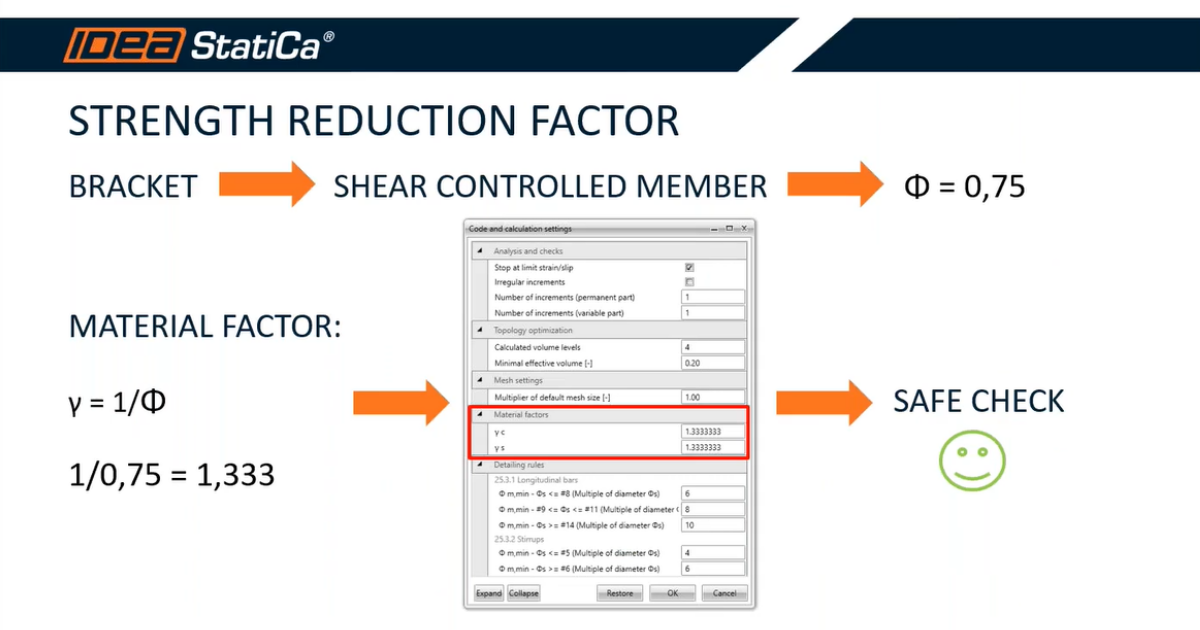### Choose language# How to input the strength reduction factor according to ACI

$$According to the code ACI we should consider a Strength reduction factor based on the type of structure we are about to analyze. In IDEA StatiCa Detail we have a Material factor, which is used to reduce the characteristic strength of concrete and reinforcement. While the Strength reduction factor is considered as a multiplier (numerator) of the material strength, the Material factor is used as a divisor (denominator). Because of that the Material factor is γ=1/Ф.$$